## 9.1 Promotions activity

Let’s start with an activity studying the effect of gender on promotions at a bank.

### 9.1.1 Does gender affect promotions at a bank?

Say you are working at a bank in the 1970s and you are submitting your résumé to apply for a promotion. Will your gender affect your chances of getting promoted? To answer this question, we’ll focus on data from a study published in the Journal of Applied Psychology in 1974. This data is also used in the OpenIntro series of statistics textbooks.

To begin the study, 48 bank supervisors were asked to assume the role of a hypothetical director of a bank with multiple branches. Every one of the bank supervisors was given a résumé and asked whether or not the candidate on the résumé was fit to be promoted to a new position in one of their branches.

However, each of these 48 résumés were identical in all respects except one: the name of the applicant at the top of the résumé. Of the supervisors, 24 were randomly given résumés with stereotypically “male” names, while 24 of the supervisors were randomly given résumés with stereotypically “female” names. Since only (binary) gender varied from résumé to résumé, researchers could isolate the effect of this variable in promotion rates.

While many people today (including us, the authors) disagree with such binary views of gender, it is important to remember that this study was conducted at a time where more nuanced views of gender were not as prevalent. Despite this imperfection, we decided to still use this example as we feel it presents ideas still relevant today about how we could study discrimination in the workplace.

The moderndive package contains the data on the 48 applicants in the promotions data frame. Let’s explore this data by looking at six randomly selected rows:

promotions %>%
sample_n(size = 6) %>%
arrange(id)
# A tibble: 6 x 3
id decision gender
<int> <fct>    <fct>
1    11 promoted male
2    26 promoted female
3    28 promoted female
4    36 not      male
5    37 not      male
6    46 not      female

The variable id acts as an identification variable for all 48 rows, the decision variable indicates whether the applicant was selected for promotion or not, while the gender variable indicates the gender of the name used on the résumé. Recall that this data does not pertain to 24 actual men and 24 actual women, but rather 48 identical résumés of which 24 were assigned stereotypically “male” names and 24 were assigned stereotypically “female” names.

Let’s perform an exploratory data analysis of the relationship between the two categorical variables decision and gender. Recall that we saw in Subsection 2.8.3 that one way we can visualize such a relationship is by using a stacked barplot.

ggplot(promotions, aes(x = gender, fill = decision)) +
geom_bar() +
labs(x = "Gender of name on résumé")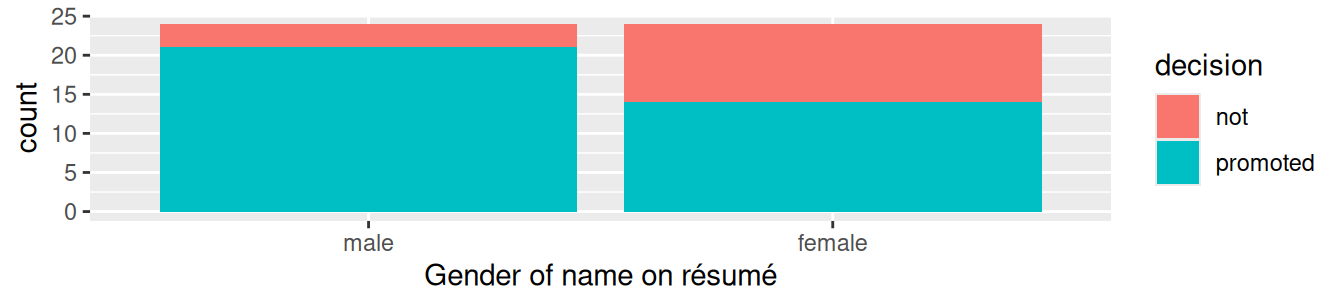FIGURE 9.1: Barplot relating gender to promotion decision.

Observe in Figure 9.1 that it appears that résumés with female names were much less likely to be accepted for promotion. Let’s quantify these promotion rates by computing the proportion of résumés accepted for promotion for each group using the dplyr package for data wrangling. Note the use of the tally() function here which is a shortcut for summarize(n = n()) to get counts.

promotions %>%
group_by(gender, decision) %>%
tally()
# A tibble: 4 x 3
# Groups:   gender 
gender decision     n
<fct>  <fct>    <int>
1 male   not          3
2 male   promoted    21
3 female not         10
4 female promoted    14

So of the 24 résumés with male names, 21 were selected for promotion, for a proportion of 21/24 = 0.875 = 87.5%. On the other hand, of the 24 résumés with female names, 14 were selected for promotion, for a proportion of 14/24 = 0.583 = 58.3%. Comparing these two rates of promotion, it appears that résumés with male names were selected for promotion at a rate 0.875 - 0.583 = 0.292 = 29.2% higher than résumés with female names. This is suggestive of an advantage for résumés with a male name on it.

The question is, however, does this provide conclusive evidence that there is gender discrimination in promotions at banks? Could a difference in promotion rates of 29.2% still occur by chance, even in a hypothetical world where no gender-based discrimination existed? In other words, what is the role of sampling variation in this hypothesized world? To answer this question, we’ll again rely on a computer to run simulations.

### 9.1.2 Shuffling once

First, try to imagine a hypothetical universe where no gender discrimination in promotions existed. In such a hypothetical universe, the gender of an applicant would have no bearing on their chances of promotion. Bringing things back to our promotions data frame, the gender variable would thus be an irrelevant label. If these gender labels were irrelevant, then we could randomly reassign them by “shuffling” them to no consequence!

To illustrate this idea, let’s narrow our focus to 6 arbitrarily chosen résumés of the 48 in Table 9.1. The decision column shows that 3 résumés resulted in promotion while 3 didn’t. The gender column shows what the original gender of the résumé name was.

However, in our hypothesized universe of no gender discrimination, gender is irrelevant and thus it is of no consequence to randomly “shuffle” the values of gender. The shuffled_gender column shows one such possible random shuffling. Observe in the fourth column how the number of male and female names remains the same at 3 each, but they are now listed in a different order.

TABLE 9.1: One example of shuffling gender variable
résumé number decision gender shuffled gender
1 not male male
2 not female male
3 not female female
4 promoted male female
5 promoted male female
6 promoted female male

Again, such random shuffling of the gender label only makes sense in our hypothesized universe of no gender discrimination. How could we extend this shuffling of the gender variable to all 48 résumés by hand? One way would be by using standard deck of 52 playing cards, which we display in Figure 9.2.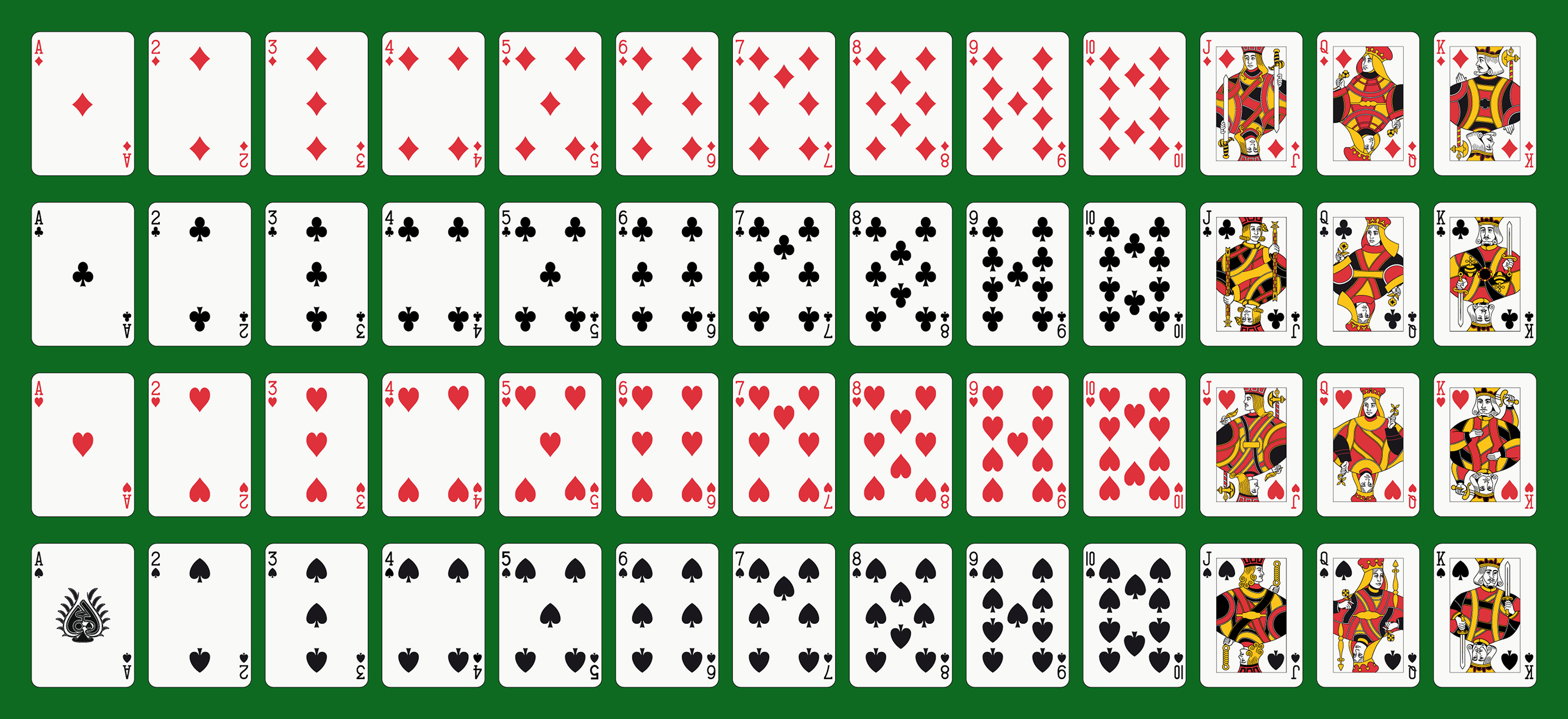FIGURE 9.2: Standard deck of 52 playing cards.

Since half the cards are red (diamonds and hearts) and the other half are black (spades and clubs), by removing two red cards and two black cards, we would end up with 24 red cards and 24 black cards. After shuffling these 48 cards as seen in Figure 9.3, we can flip the cards over one-by-one, assigning “male” for each red card and “female” for each black card.FIGURE 9.3: Shuffling a deck of cards.

We’ve saved one such shuffling in the promotions_shuffled data frame of the moderndive package. If you compare the original promotions and the shuffled promotions_shuffled data frames, you’ll see that while the decision variable is identical, the gender variable has changed.

Let’s repeat the same exploratory data analysis we did for the original promotions data on our promotions_shuffled data frame. Let’s create a barplot visualizing the relationship between decision and the new shuffled gender variable and compare this to the original unshuffled version in Figure 9.4.

ggplot(promotions_shuffled,
aes(x = gender, fill = decision)) +
geom_bar() +
labs(x = "Gender of résumé name")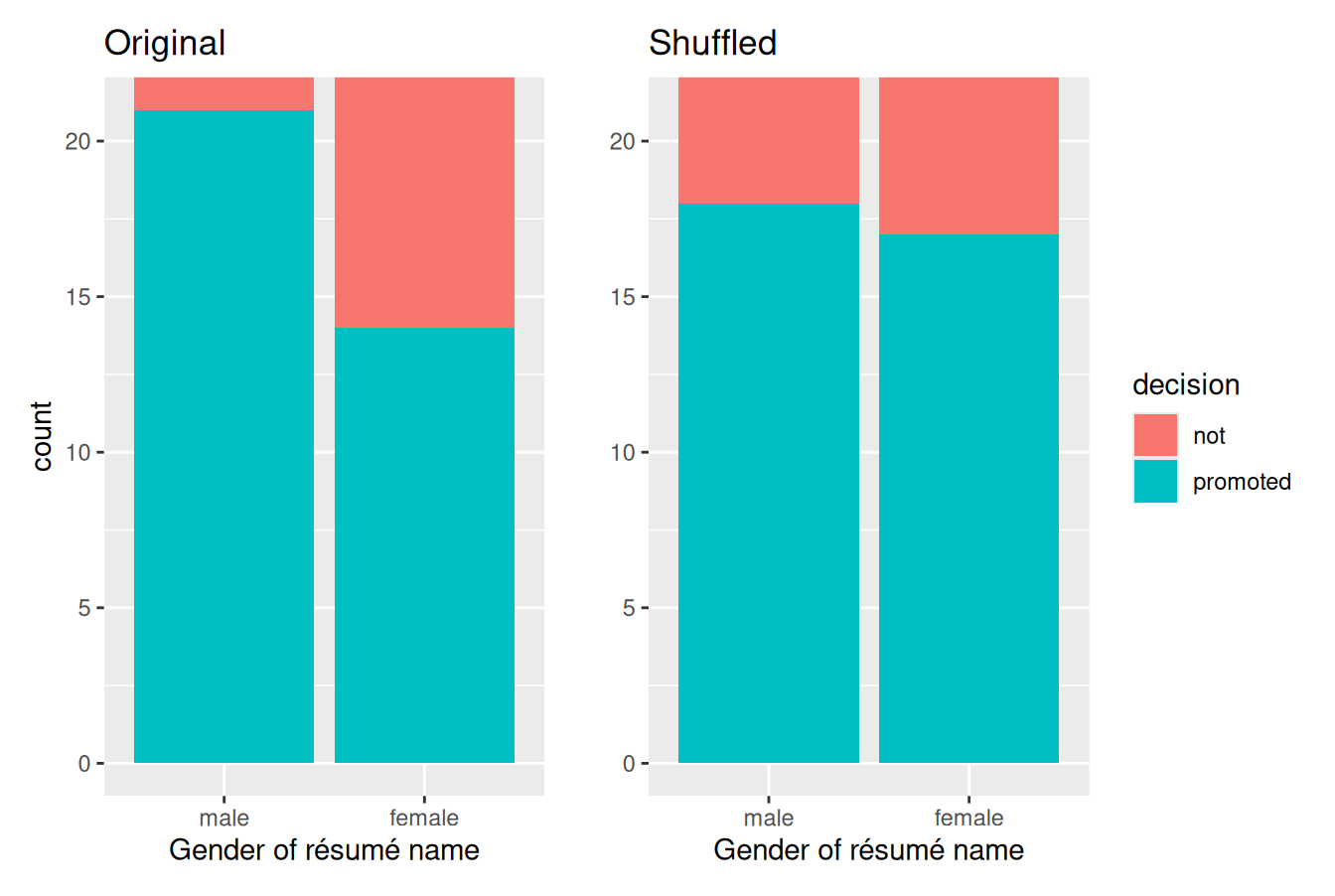FIGURE 9.4: Barplots of relationship of promotion with gender (left) and shuffled gender (right).

It appears the difference in “male names” versus “female names” promotion rates is now different. Compared to the original data in the left barplot, the new “shuffled” data in the right barplot has promotion rates that are much more similar.

Let’s also compute the proportion of résumés accepted for promotion for each group:

promotions_shuffled %>%
group_by(gender, decision) %>%
tally() # Same as summarize(n = n())
# A tibble: 4 x 3
# Groups:   gender 
gender decision     n
<fct>  <fct>    <int>
1 male   not          6
2 male   promoted    18
3 female not          7
4 female promoted    17

So in this hypothetical universe of no discrimination, $$18/24 = 0.75 = 75\%$$ of “male” résumés were selected for promotion. On the other hand, $$17/24 = 0.708 = 70.8\%$$ of “female” résumés were selected for promotion.

Let’s next compare these two values. It appears that résumés with stereotypically male names were selected for promotion at a rate that was $$0.75 - 0.708 = 0.042 = 4.2\%$$ different than résumés with stereotypically female names.

Observe how this difference in rates is not the same as the difference in rates of 0.292 = 29.2% we originally observed. This is once again due to sampling variation. How can we better understand the effect of this sampling variation? By repeating this shuffling several times!

### 9.1.3 Shuffling 16 times

We recruited 16 groups of our friends to repeat this shuffling exercise. They recorded these values in a shared spreadsheet; we display a snapshot of the first 10 rows and 5 columns in Figure 9.5.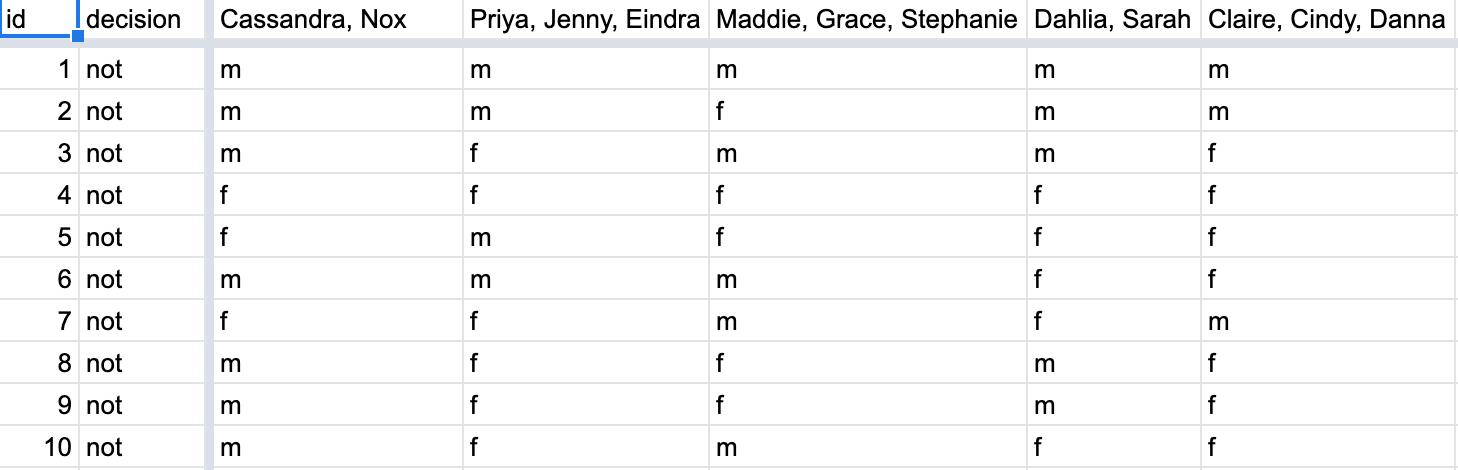FIGURE 9.5: Snapshot of shared spreadsheet of shuffling results (m for male, f for female).

For each of these 16 columns of shuffles, we computed the difference in promotion rates, and in Figure 9.6 we display their distribution in a histogram. We also mark the observed difference in promotion rate that occurred in real life of 0.292 = 29.2% with a dark line.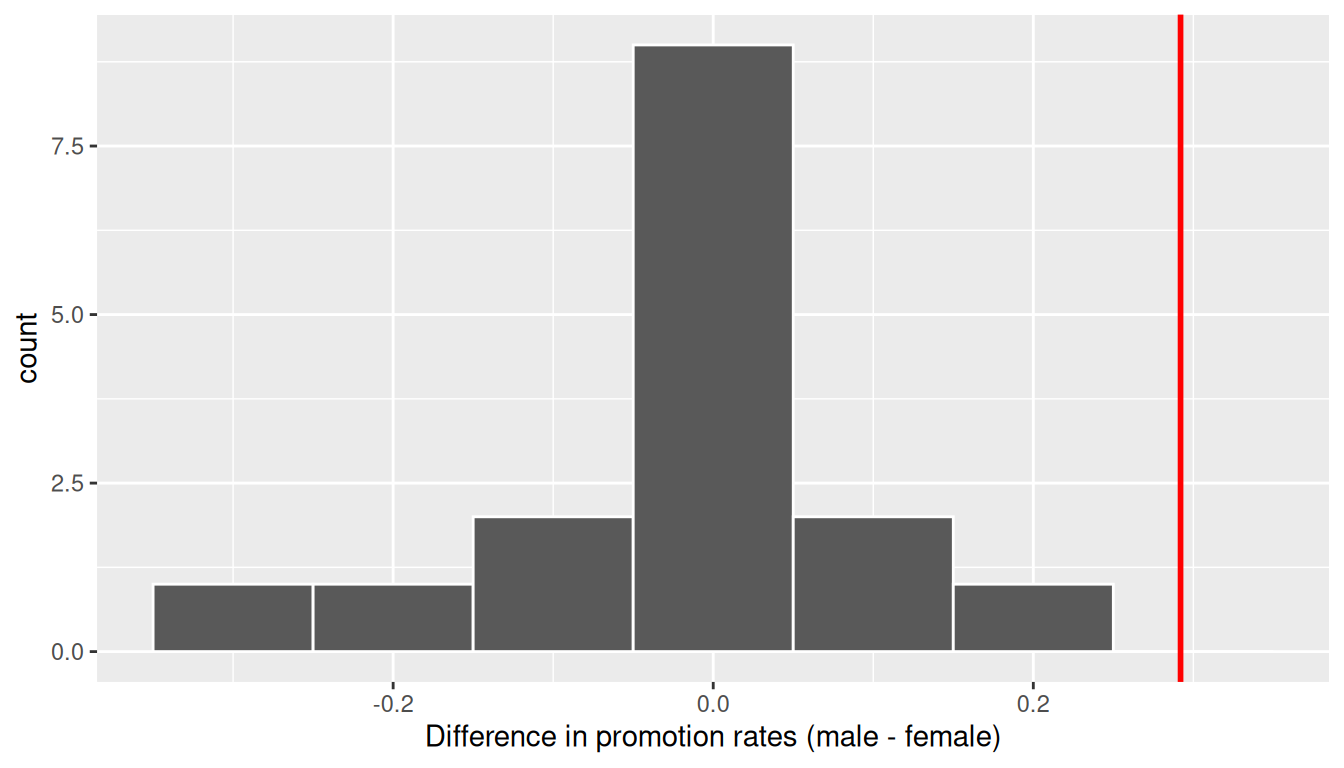FIGURE 9.6: Distribution of shuffled differences in promotions.

Before we discuss the distribution of the histogram, we emphasize the key thing to remember: this histogram represents differences in promotion rates that one would observe in our hypothesized universe of no gender discrimination.

Observe first that the histogram is roughly centered at 0. Saying that the difference in promotion rates is 0 is equivalent to saying that both genders had the same promotion rate. In other words, the center of these 16 values is consistent with what we would expect in our hypothesized universe of no gender discrimination.

However, while the values are centered at 0, there is variation about 0. This is because even in a hypothesized universe of no gender discrimination, you will still likely observe small differences in promotion rates because of chance sampling variation. Looking at the histogram in Figure 9.6, such differences could even be as extreme as -0.292 or 0.208.

Turning our attention to what we observed in real life: the difference of 0.292 = 29.2% is marked with a vertical dark line. Ask yourself: in a hypothesized world of no gender discrimination, how likely would it be that we observe this difference? While opinions here may differ, in our opinion not often! Now ask yourself: what do these results say about our hypothesized universe of no gender discrimination?

### 9.1.4 What did we just do?

What we just demonstrated in this activity is the statistical procedure known as hypothesis testing using a permutation test. The term “permutation” is the mathematical term for “shuffling”: taking a series of values and reordering them randomly, as you did with the playing cards.

In fact, permutations are another form of resampling, like the bootstrap method you performed in Chapter 8. While the bootstrap method involves resampling with replacement, permutation methods involve resampling without replacement.

Think of our exercise involving the slips of paper representing pennies and the hat in Section 8.1: after sampling a penny, you put it back in the hat. Now think of our deck of cards. After drawing a card, you laid it out in front of you, recorded the color, and then you did not put it back in the deck.

In our previous example, we tested the validity of the hypothesized universe of no gender discrimination. The evidence contained in our observed sample of 48 résumés was somewhat inconsistent with our hypothesized universe. Thus, we would be inclined to reject this hypothesized universe and declare that the evidence suggests there is gender discrimination.

Recall our case study on whether yawning is contagious from Section 8.6. The previous example involves inference about an unknown difference of population proportions as well. This time, it will be $$p_{m} - p_{f}$$, where $$p_{m}$$ is the population proportion of résumés with male names being recommended for promotion and $$p_{f}$$ is the equivalent for résumés with female names. Recall that this is one of the scenarios for inference we’ve seen so far in Table 9.2.

TABLE 9.2: Scenarios of sampling for inference
Scenario Population parameter Notation Point estimate Symbol(s)
1 Population proportion $$p$$ Sample proportion $$\widehat{p}$$
2 Population mean $$\mu$$ Sample mean $$\overline{x}$$ or $$\widehat{\mu}$$
3 Difference in population proportions $$p_1 - p_2$$ Difference in sample proportions $$\widehat{p}_1 - \widehat{p}_2$$

So, based on our sample of $$n_m$$ = 24 “male” applicants and $$n_w$$ = 24 “female” applicants, the point estimate for $$p_{m} - p_{f}$$ is the difference in sample proportions $$\widehat{p}_{m} -\widehat{p}_{f}$$ = 0.875 - 0.583 = 0.292 = 29.2%. This difference in favor of “male” résumés of 0.292 is greater than 0, suggesting discrimination in favor of men.

However, the question we asked ourselves was “is this difference meaningfully greater than 0?”. In other words, is that difference indicative of true discrimination, or can we just attribute it to sampling variation? Hypothesis testing allows us to make such distinctions.